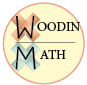WM Fraction System
The version of the browser you are using is no longer supported. Please upgrade to a supported browser.Dismiss

1
2Vertical Path of Skill Development for the WoodinMath Fraction System
3
4
5
6
7
8
Readiness Skills: Whole-To-Part Relationships
Article:
Durable Images Teach Fractions
9
Stage 1: Symmetry Within Wholes (partitioning into equal halves) - Kindergarten
10
This stage will help children develop an appreciation of symmetry within common objects. Common objects are named. Basic shapes and solids are labeled and divided in two by area, volume and mass. Children will identify pairs of items to make a whole unit (shoes, mittens, ears).
11
12
13
Conceptual Models
Numeration
Comparison
Facts
Procedures
Applications
14
Look for and make use of structure.
15
Semantics driving quantity
Quantity driving Semantics
16
2= 1+1 4=2 +2
17
Complete the pair using language
Complete the Pair using visual matching
18
Wholes and named attributes
19
Spatial symmetry
Pop Bubbles to Define Shape AttributesClassify Polygons by Attributes using Pipe manipulatives
20
K.G.3 Identify and describe shapes
📽 Related Video of Shape Construction and ClassificationShape Name Vocabulary Notes and worksheets
21
22
Evaluation: Necessary pre-requisite skills to move to Stage 2: the ability to perform the above activities with ample wait time, but minimal external cueing.
23
Stage 2: Attributes Within Wholes (partitioning wholes into same-sized groups)
24
This stage will help children apportion common objects into two or more equal subordinate parts (attributes). Basic shapes are labeled and divided along axes of symmetry. Coins are named. Students will decompose whole numbers into same sized-parts and forge related number statements.
25
Conceptual Models
Numeration
Comparison
Facts
Procedures
Applications
26
even numbersDecompose quantities less than or equal to 12 into same-sized addends in more than one way, e.g., by using objects or drawings, and record each decomposition by a drawing or equation (e.g., 6 = 3 + 3 and 6 = 2 + 2 + 2, and 6 =1+1+1+1+1+1).Identify whether the number of objects in one group is greater than, less than, or equal to the number of objects in another group for groups with up to 10 objects, e.g., by using matching and counting strategies.
27
28
Encode Fraction UniverseInitial Fraction Universe Encoding Model Durable Image Fraction Models
29
30
31
Stage 3: Fraction Addition and Subtraction (like denominators)
32
In this stage, students add and subtract (parts) attributes of the same whole using fractions and mixed numbers with common denominators.
33
Conceptual Models
Numeration
Comparison
Facts
Procedures
Applications
34
35
Add Type 1 Fractions with Common Denominators and Durable ImagesAdd and Subtract Fractions Type 1 Students Encode, then Set-up Problems
36
37
38
39
40
Evaluation: Necessary pre-requisite skills to move to Stage 2: the ability to perform the above activities with ample wait time, but minimal external cueing.
41
Stage 4: Fraction Equivalence and Related Operations
42
In this stage, students learn to rename fractions to equivalent forms based on attributes ( 1 left hand:1/2 = 5 left fingers: 5/10) and then perform this procedurally. Decimal fractions are equated to decimal numbers within the hundredths place and linked to currency. Fractions and mixed numbers with unlike denominators are added and subtracted.
43
Conceptual Models
Numeration
Comparison
Facts
Procedures
Applications
44
45
Fraction Frame Template
46
Recognize and generate simple equivalent fractions, e.g., 1 ∕2 = 2 ∕4, 4 ∕6 = 2 ∕3. Explain why the fractions are equivalent, e.g., by using a visual fraction model.Fraction Universe fractions equivalent to 1/2 movieAll Student Respond = Fractions Durable ImagesRenaming Equivalent Fractions using Durable ImagesExpand and Simplify Fractions While Practicing Fact Families and the Multiplication Table Area Model📽 Kinesthetic Line plot of fractions 1/2 and 4ths internal reference frame📽 Kinesthetic Line plot of fractions within an inch external reference frame
47
48
Fraction Graphic Organizer Cards and Templates to Rename and add 📽Video for Fraction Graphic Organizer Cards and Templates to Rename and add Ant Cards Plot diagrams and fractions on number lineFind Mixed Numbers on Ruler DiagramsPerimeter Fractions
49
📽 Add Type 2 Fractions using Semantic Cues to Rename FractionsType 1 and 2 Addition and Subtraction C.D.= 6 using 6-Pack GraphicType 1 and 2 Addition and Subtraction C.D.= 8 using Octopus GraphicAdd and subtract Type 2 with scaffolded Semantic DiagramsDetermine Common Multiple Type 1,2,3
50
Tactile Currency WorksheetEncode Decimal FractionsSplit between two fraction values worksheetAdd Decimal Fractions and decimals relating to money in Tandem
51
📽1/4 Inch Ruler Warm-up Drill
52
📽 Convert improper fractions to mixed numbers with Fraction Universe modelRegroup Mixed # IntroductionImproper to mixed number worksheet series.Mixed # Regroup Semantic DiagramTree Bud Measurement Tree Bud Measurement Video
53
Mixed Number addition and subtraction with linear diagrams
54
55
56
57
58
59
60
Stage 5: Multiplying and Dividing Fractions and Mixed Numbers and Fraction / Decimal /Percent Conversion
61
In this stage,
62
63
Multiply whole numbers by 1/2 three Ways:Multiply whole numbers by 1/10 three waysMultiply whole numbers by 1/4 three waysMultiply whole numbers by 1/5 three waysWhole number x unit fraction word problems
64
65
66
67
68
69
70
71
72
73
74
75
76
77
78
79
80
81
82
83
84
85
86
87
88
89
90
91
92
93
94
95
96
97
98
99
100Loading...